#Interactive Real Analysis

Next | Previous | Glossary | Map

## 8.1. Pointwise Convergence

### Example 8.1.8 (e): Pointwise Convergence does not preserve Integration

Find a pointwise convergent sequence of Riemann-integrable functions whose limit is Riemann-integrable but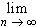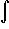fn(x) dx #fn(x) dx =f(x) dx

 This is perhaps a little tricky, but we could try one of the function sequences we discussed earlier: fn(x) = max(n - n2 |x - 1/n|, 0) where x[ 0, 1 ] (you knew this function would play a bigger role, didn't you). Recall that each member of this family is piecewise linear, so another way to write fn is:Each fn is continuous, hence Riemann integrable. The pointwise limit f is zero, which is again integrable. But when we integrate: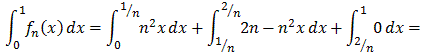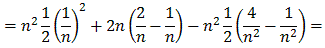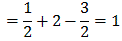for all n. On the other hand, the integral of the limit function is clearly zero so that:
1 =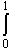fn(x) dx #f(x) dx = 0
By the way, if we consider the integral as area under a function it is immediately clear from the graph that the fn's integrate to 1 ...
Next | Previous | Glossary | Map